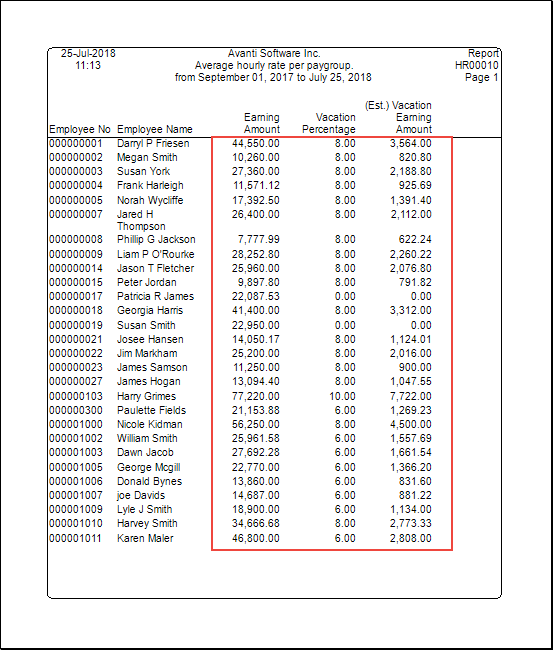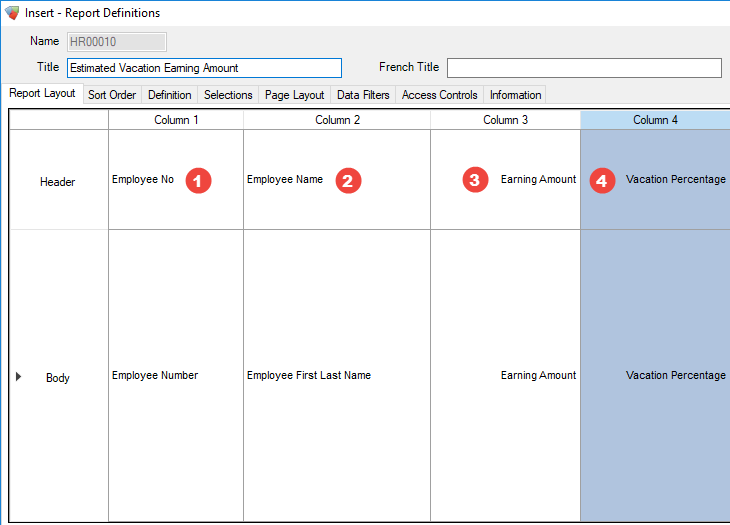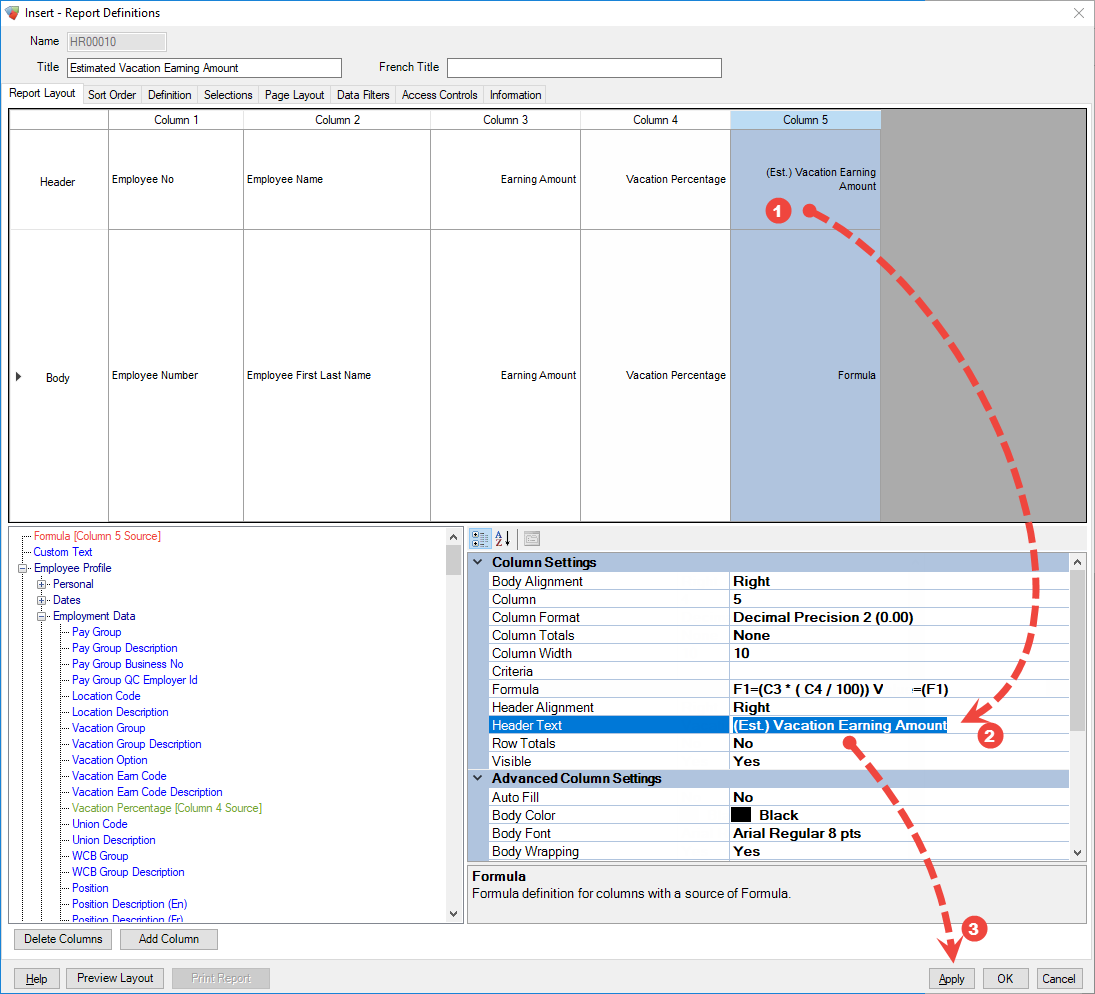# Creating a Formula Driven Report

Formulas are one of the more-powerful features available within Report Designer. Using formulas you can perform calculations using data from other columns, and create unique results that would otherwise be impossible using just the sources alone.

This document will teach you how to
• Use C-Variables to pull data from other columns into formulas.

• Rename the column header used when printing a report.

We will go over the steps to create a new Report Definition that will calculate an estimate of the Vacation Earnings amount for each employee.Figure 1. Example of what the report we will create looks like when printed.

## Create Report Definition

The report we will be making will contain five columns—four which will use data sources, and the last column will use a formula to calculate the Vacation Earning Amount of each employee.

 Adding columnsYou can add columns to the report a couple of ways:DraggingYou can drag a source directly onto the dark-gray area of the Report Layout columns to create a new column using that source. (If you drag the source onto a column you will be prompted to replace that column’s source however.)Right-click MenuYou can right-click on the column area to open a right click menu where you can chose to add a column, or insert a new column before or after the column which is currently selected.
1. Add the following columns to the report:Figure 2. The four columns using data-source for this report.
Sources
 1 `Employee Profile > Personal > Employee Number` 2 `Employee Profile > Personal > Employee Name` 3 `History > Pay Period > Earning Amount` 4 `Employee Profile > Employment Data > Vacation Percentage`
2. Add a formula column to the report.

We’ll go over the steps to setup the formula column with a formula that will calculate the Vacation Earning Amount in the following section.

## Open the Formula Settings

Before we setup the formula, you will need to open the formula settings window for the formula column.

You can open the formula settings using these steps:
1. Select the formula column.

2. From the Properties Panel select the `Formula` property.

3. Press the […] button to open the formula.Figure 3. Steps to open the Column Formula settings.

## Formula Setup

The formula that we are going to use looks like this:

Formula:
```Formulas F1 = C3 * (C4 / 100) (1)
Value = F1 (2)```
 1 The Vacation Percent is converted to a decimal here to make the result correct. 2 The result from `F1` is used as the value for the column data.

What the formula is doing, in plain English, is converting the Vacation Percentage to a decimal then multiplying the Earning Amount column by the decimal result to calculate the Vacation Earning Amount.

 Note:ParenthesisThe parenthesis in the above formula are used to infer the order that the calculation will occur.
Enter the formula into the column formula setup:
1. Enter `C3 * (C4 / 100))` into the `Formulas F1` field.

2. Enter `F1` into the `Value` field.

3. Save the formula by pressing now.Figure 4. Example of the Column Formula setup using the formula.

## C-Variables

In the formula above we used two C-Variables (`C3`, and `C4`) to bring data into the formula from other columns.

• Each C-Variables refers to the value of the current row from the numbered column.

• `Column 1` would be referenced using the `C1` C-Variables. (The column numbers are viewable above the column headers in the Report Definition.)

## Rename the Formula Column

The final thing that you may want to adjust being printing the report is to change the header text of the Formula Column. Right now the formula column header will be set to Formula, but this should be changed to something more meaningful.

1. Select the formula column.

2. From the Column Properties select Column Settings > Header Text and enter a new value to use for the column header.

3. Press Apply to save the changes to the report definition.

 Note: These steps will work to rename the header of any column, not only the formula column.Figure 5. Steps required to change the Header Text of a column.

## Example of the Completed Report

After you have setup the Report Definition with a formula column you will want to preview the report. The following is an example of what the report may look like when it is run.Figure 6. Example of the completed report printed using A5 paper.
 1 The values from the Earning Amount column (Column 3) are referenced using the C3 C-Variables 2 The values from the Vacation Percentage column (Column 4) are referenced using the C4 C-Variables 3 The formula column uses the formula we setup to calculate the Vacation Earning Amount from each row of Column 3 and Column 4. (Note that the formula column uses the header text we entered previously.)

Did you find it helpful? Yes No

Send feedback